Subsections

# Relaxation & Time Correlation Function

## Relaxation Time

Suppose we have a thermodynamic variable x with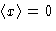. What happens if at some moment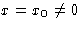?

Thermodynamics Language:
We have a restricted ensemble: a set of systems with the given x.
Example:
I prepared a set of beakers at the temperature T0 and put it in the thermostat with the temperature T.
Kinetics and Equilibrium:
A beaker at the temperature T is not in the equilibrium with the environment--technically speaking, we cannot use thermodynamics!
A Trick:
we say that it is partially equilibrated--everything is equilibrated for this temperature.
General situation:
we have a slowly changing variable x, and say, that every other degree of freedom is fast.
Then we have a function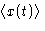, such as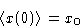(1)

Atwe have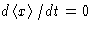(why?). For small deviations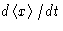is small. We can expand it in series in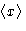, and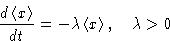(2)
Solution: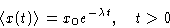(3)
Small fluctuations decay exponentially with the relaxation time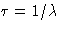.

This formula is valid only for t>0: we can prepare an ensemble with given x0 and observe it, but cannot go backward! This is another manifestation of time irreversibility.

## Time Correlation Function

For space-dependent problems we defined the correlation function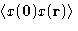. For time-dependent case we define time correlation function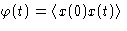. We want to calculate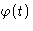. This is the averaging over the ensemble of systems with arbitrary x(0). Note this difference with the previous case: there we had x(0) fixed, and used restricted ensembles. Now we return to the general case and consider equilibrium ensemble.

Translation along the time axis: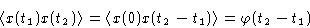and therefore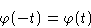(4)

Double averaging method:

1.
Divide the ensemble into subensembles with given x(0)=xi. Each subensemble has many microscopic states with given macroscopic value x(0).
2.
Average x(0)x(t) in a subensemble: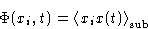3.
Average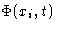over all subensembles: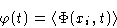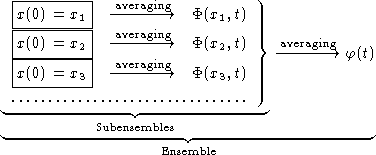Since in a subensemble x(0) is given,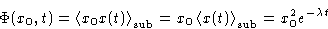Second averaging: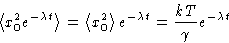with generalized susceptibility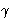.

We obtained for the correlation function: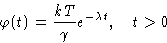From (4) we obtain for negative t that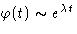, or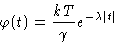(5)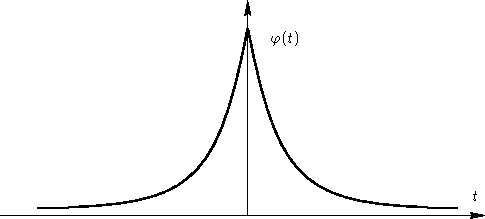This function has different derivatives at: time irreversibility!

## Multi-Variate Case

### Kinetic Equations

We have several variables xi. Kinetic equations: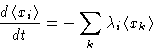Now we have multi-variate correlation functions: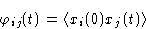### Symmetry properties

1.
Translation of the time axis: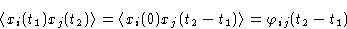We see: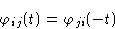(6)
2.
Equations of motion are symmetric with respect to time inversion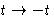: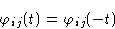(7)
3.
From (6) and (7)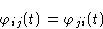(8)Next: Thermodynamic Forces & Kinetic Up: Time Dependent Fluctuations Previous: Time Dependent Fluctuations

© 1997 Boris Veytsman and Michael Kotelyanskii
Tue Oct 28 22:13:33 EST 1997